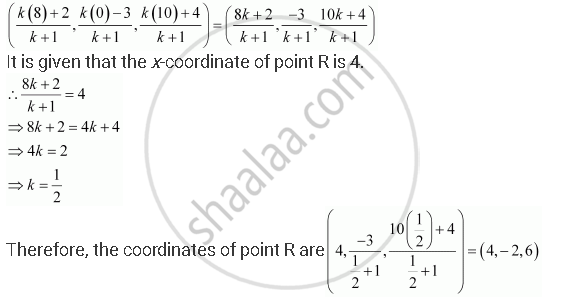CBSE (Arts) Class 11CBSE
Share

# A Point R With X-coordinate 4 Lies on the Line Segment Joining the Pointsp (2, –3, 4) and Q (8, 0, 10). Find the Coordinates of the Point R. - CBSE (Arts) Class 11 - Mathematics

ConceptThree - Dimensional Geometry Coordinates of a Point in Space

#### Question

A point R with x-coordinate 4 lies on the line segment joining the pointsP (2, –3, 4) and Q (8, 0, 10). Find the coordinates of the point R.

[Hint suppose R divides PQ in the ratio k: 1. The coordinates of the point R are given by ((8k + 2)/(k+1), (-3)/(k+1), (10k + 4)/(k+1))

#### Solution

The coordinates of points P and Q are given as P (2, –3, 4) and Q (8, 0, 10).

Let R divide line segment PQ in the ratio k:1.

Hence, by section formula, the coordinates of point R are given byIs there an error in this question or solution?

#### APPEARS IN

NCERT Solution for Mathematics Textbook for Class 11 (2018 to Current)
Chapter 12: Introduction to Three Dimensional Geometry
Q: 5 | Page no. 279

#### Video TutorialsVIEW ALL 

Solution A Point R With X-coordinate 4 Lies on the Line Segment Joining the Pointsp (2, –3, 4) and Q (8, 0, 10). Find the Coordinates of the Point R. Concept: Three - Dimensional Geometry - Coordinates of a Point in Space.
S